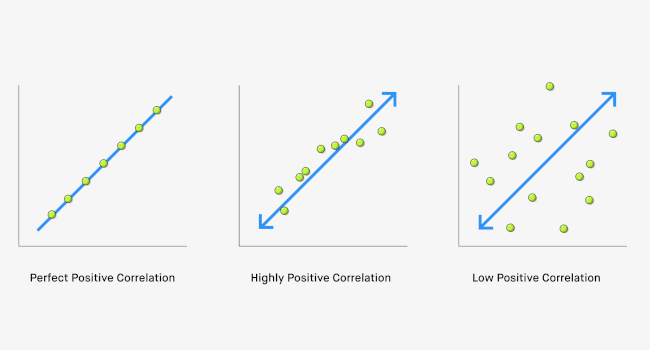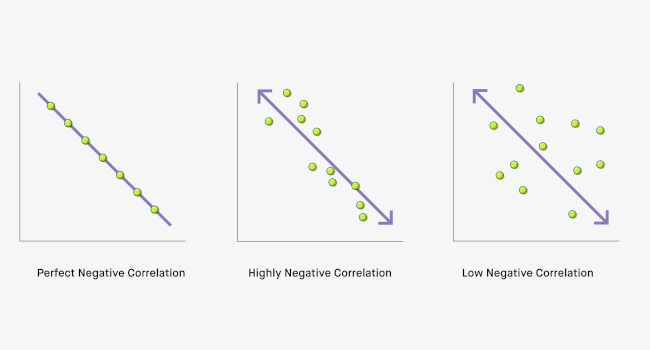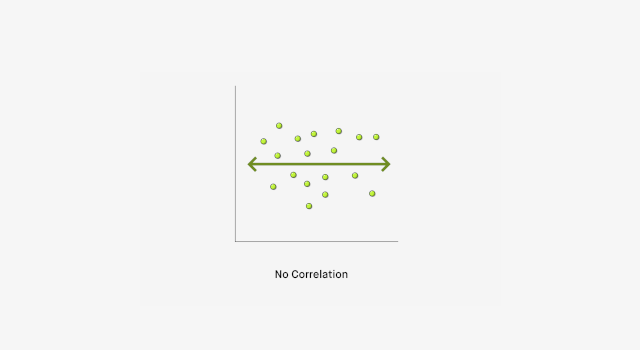# What are Correlation Coefficients?

## The ultimate guide to correlation coefficientsA correlation coefficient is the statistical measure that will tell us whether there is a relationship between our two variables of interest, and if there is one, how strong that relationship is. The value of the correlation coefficient, ρ (rho), ranges from -1 to +1. The closer to -1 or +1, the stronger the relationship is. We've also prepared a guide to nominal, ordinal, interval, ratio scales.

### What is a correlational study?

The purpose of a correlational study is to examine the potential relationship between two variables. In this type of study design, researchers will quantify two variables of relevance to their research question, and then statistically determine if the two variables are related to one another.

For a correlational study, we may ask research questions such as:

1. Is there a relationship between the number of cigarettes smoked per day and the likelihood of developing lung cancer?
2. Is there a relationship between the number of hours spent exercising per week and levels of depression?
3. Is there a relationship between the color of your shirt and the score you receive on a mathematics exam?

### What is a positive correlation?

When ρ is close to +1, this tells us that there is a positive, or direct, relationship between the two variables. This means that as one variable increases, the second variable also increases. Consider our first example: let's assume that there is a positive relationship between the number of cigarettes smoked per day and the likelihood of developing lung cancer. This means that as the number of cigarettes smoked per day increase, the chances of developing lung cancer also increase.### What is a negative correlation?

In contrast, when ρ is close to -1, this tells us that there is a negative, or inverse, relationship between the two variables. This means that as one variable increases, the second variable decreases. Consider our second example: let's assume that there is an inverse relationship between the number of hours spent exercising and levels of depression. This means that as the number of hours spent exercising increases, levels of depression decrease. With a negative correlation, this could also be interpreted the opposite way: as the number of hours spent exercising decrease, levels of depression increase.

### When there is no correlation

Alternatively, when ρ is close to 0, this means that there is a weak, or no, relationship between the two variables. This means that our two variables are likely not related to one another. Consider our third example: let's assume that there is no correlation between the color of your shirt and the score you receive on a mathematics exam. This means that whichever shirt you wear has nothing to do with how well you do on the test.### Correlational Study Designs

With a correlational study design, we wish to determine if there is a relationship between two variables. We therefore need to consider the following when designing our study:

1. What our hypotheses are: if we wish to examine if there is a relationship between two variables, we need to base our predictions on existing research. For a correlation, we would therefore hypothesise that:
a. Null hypothesis: the correlation between the two variables is 0 (there is no relationship between the variables of interest); or
b. Alternate hypothesis: the correlation between the two variables is not 0 (there is a relationship between the variables of interest).
2. Inclusion of two quantitative and continuous variables: this means that both variables can have any numerical value, and should not be discrete (i.e. categorical). The two variables would be based on what relationship we want to look at.
3. How we are measuring our variables: once we decide which variables we are investigating, we need to determine how they are going to be measured/quantified. How this is done depends on the variable of interest. For example, if we were measuring the number of hours of exercise, this could be a number recorded each day for a certain period. For depression levels, this could be quantified using a questionnaire. Another option is to use data that has already been collected (referred to as archival data).
4. Determining a between or within-subjects design: we then need to decide who the variables are being measured in.This means that for our correlational study:
a. Both variables should be measured on the same person or group of people (within-subjects); or
b. We have one variable measured in one group, and the other variable measured in another (between-subjects).

Once we have designed our correlational study, collected our data and calculated the correlation coefficient, we can then conclude if there is a relationship between our two variables, and if there is one, what kind of relationship it is (positive, negative, or none; and how strong that relationship is).

### Correlation does not equal causation

It's important to remember that a correlation will only tell us if there is a relationship between our two variables. However, correlation does not equal causation. Based on a correlation, we cannot infer that one variable causes the change (increase or decrease) in the other, but we can only see that a relationship exists.

### Dynamic Correlations

Another key factor is that correlations can be dynamic. A relationship between two variables may be present now, however it's not set in stone. For example, a positive correlation may become negative or zero in future studies due to a variety of factors, such as different sample sizes, measurement of the two variables in different groups (e.g. measuring them in the elderly instead of teenagers), measurement of the two variables using different approaches (e.g. changing questionnaires), and so on. This is what makes research interesting - seeing how relationships change!